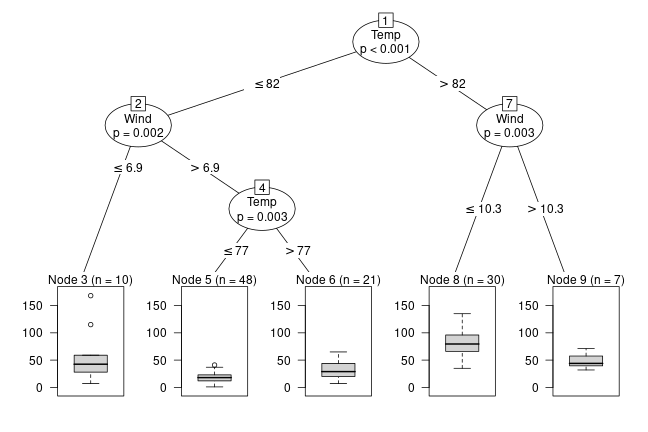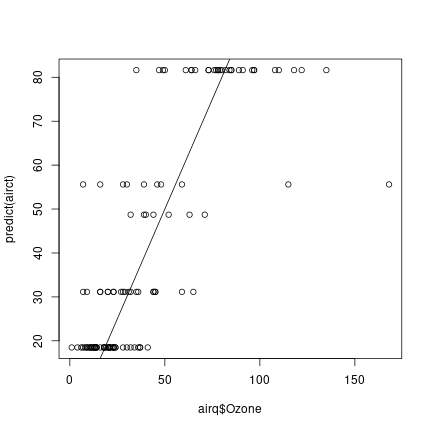First, install and fire-up R on your computer. Within R, one needs to install the party package by typing

install.packages("party")


and hitting the ENTER key. Once the package is installed, you can load it using

library("party")

## Loading required package: grid

## Loading required package: mvtnorm

## Loading required package: modeltools

## Loading required package: stats4

## Loading required package: strucchange

## Loading required package: zoo

##
## Attaching package: 'zoo'

## The following objects are masked from 'package:base':
##
##     as.Date, as.Date.numeric

## Loading required package: sandwich


Now all party functions are ready to be used, for example the ctree() function for fitting a regression tree to the Ozone data (after removing observations with missing response):

### regression
airq <- subset(airquality, !is.na(Ozone))
airct <- ctree(Ozone ~ ., data = airq,
controls = ctree_control(maxsurrogate = 3))
airct

##
##   Conditional inference tree with 5 terminal nodes
##
## Response:  Ozone
## Inputs:  Solar.R, Wind, Temp, Month, Day
## Number of observations:  116
##
## 1) Temp <= 82; criterion = 1, statistic = 56.086
##   2) Wind <= 6.9; criterion = 0.998, statistic = 12.969
##     3)*  weights = 10
##   2) Wind > 6.9
##     4) Temp <= 77; criterion = 0.997, statistic = 11.599
##       5)*  weights = 48
##     4) Temp > 77
##       6)*  weights = 21
## 1) Temp > 82
##   7) Wind <= 10.3; criterion = 0.997, statistic = 11.712
##     8)*  weights = 30
##   7) Wind > 10.3
##     9)*  weights = 7


The tree is represented by an object called airct which can be plotted

plot(airct)or used for computing predictions

summary(predict(airct))

##      Ozone
##  Min.   :18.48
##  1st Qu.:18.48
##  Median :31.14
##  Mean   :42.13
##  3rd Qu.:81.63
##  Max.   :81.63


which can be compared to the actual response values:

plot(airq\$Ozone, predict(airct))
abline(a = 0, b = 1)